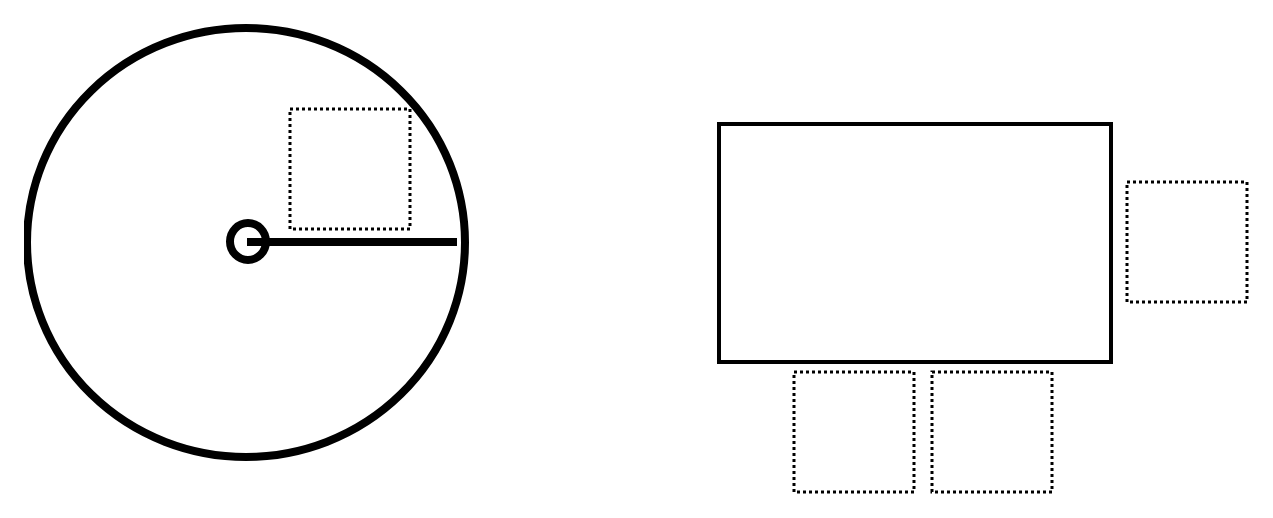# Square and Circle Area

Directions: Find the largest combined area for the rectangle and circle by filling the boxes with numbers 1 through 6. You may use a digit at most once.### Hint

Which numbers would yield a greater area?
Which numbers would yield the greatest area for just the rectangle?
Which number would yield the greatest area for the just the circle?
How can you combine these to find the greatest combined area?

The largest area is found by having a circle with radius of 4 and the dimensions of the rectangle are 6 by 53. Total area is about 368.24 square units.

Source: Mike Chamberlain

## Similar Triangles And Slope

Directions: The three triangles on the line are similar. Using the digits 0 to 9 …

1.I was experimenting and found the lowest number possible. With a radius of 3, and base of 24, and a height of 1, I ended up with a 52.27433388.

•One of my students came up something lower. A radius of 2, base of 34, and height of 1 makes an area of 46.56.

2.Should this be titled “Rectangle and Circle Area” instead? Some of my students were mislead and thought the rectangle had to be a square.

•I just created a document to share with my students & made that exact change.

3.Ditto Zach’s comment – you should change the name.